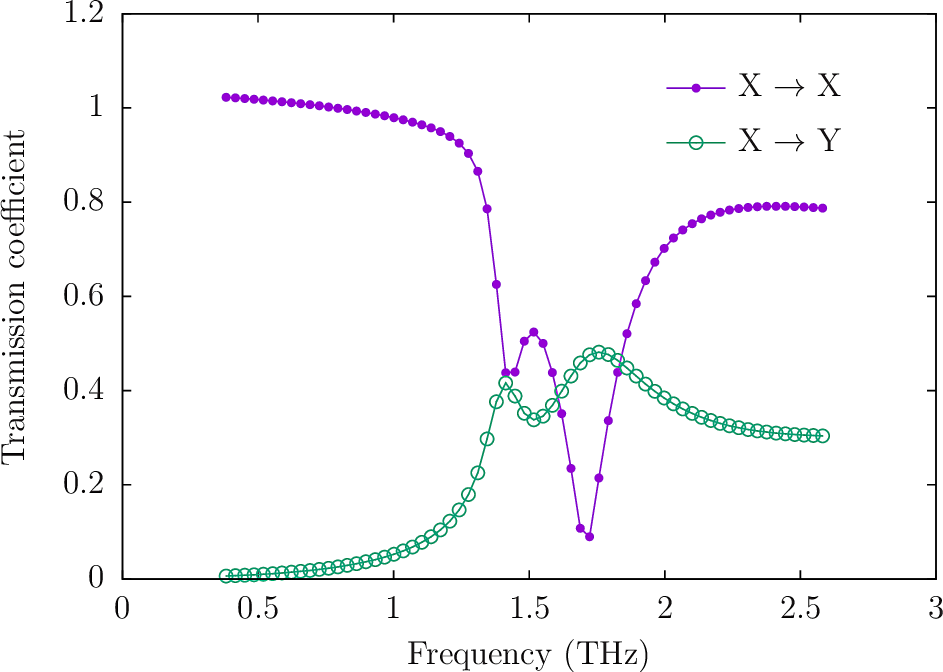# Polarization-rotated transmission through a chiral metasurface

In this example we study the phenomenon reported in this paper:

• Yi-Ju Chiang and Ta-Jen Yan, "A composite-metamaterial-based terahertz-wave polarization rotator with an ultrathin thickness, an excellent conversion ratio, and enhanced transmission", Applied Physics Letters 102 011129 (2013). DOI: http://dx.doi.org/10.1063/1.4774300

The idea is to construct a chiral metasurface consisting of an infinite planar array of scatterers with definite handedness, with the scatterers here consisting of thin metal strips in specific patterns. Over a certain range of wavelengths, shining x-polarized light on the structure from below preferentially yields a flux of y-polarized light into the upper half-space.

The files for this example may be found in the ChiralMetasurfaceRotator subdirectory of the SCUFFTutorial archive.

### GMSH geometries and meshes for asymmetric split-ring resonator (ASRR) and S-shaped resonator (SR)

The actual structure described in the paper of Chiang and Yan consists of finite (200 nm)-thickness copper traces on either side of a 50 $\mu$m-thick dielectric substrate. For this demonstration we will slightly idealize this geometry by (a) taking the traces to be infinitely thin and perfectly conducting, (b) taking the dielectric constant of the substrate to be unity. That is, our geometry will consist of infinitesimally thin PEC sheets suspended in vacuum.

## GMSH Geometry and mesh files for asymmetric split-ring resonator (ASRR) and S-shaped resonator (SR)

ASRR.geo

DefineConstant[ A = 30 ];
DefineConstant[ B = 7  ];
DefineConstant[ C = 2  ];
DefineConstant[ G = 4  ];
DefineConstant[ W = 4  ];

DefineConstant[ L = 1  ];

Point(1)  = { C,     B,         0, L  };
Point(2)  = { C+A,   B,         0, L  };
Point(3)  = { C+A,   B+A,       0, L  };
Point(4)  = { C,     B+A,       0, L  };
Point(5)  = { C,     B+A-W,     0, L  };
Point(6)  = { C+A-W, B+A-W,     0, L  };
Point(7)  = { C+A-W, B+W,       0, L  };
Point(8)  = { C+W,   B+W,       0, L  };
Point(9)  = { C+W,   B+A-W-G,   0, L  };
Point(10) = { C,     B+A-W-G,   0, L  };

Line(1) =  {1,2};
Line(2) =  {2,3};
Line(3) =  {3,4};
Line(4) =  {4,5};
Line(5) =  {5,6};
Line(6) =  {6,7};
Line(7) =  {7,8};
Line(8) =  {8,9};
Line(9) =  {9,10};
Line(10) = {10,1};

Line Loop(1)={1,2,3,4,5,6,7,8,9,10};
Ruled Surface(1)={1};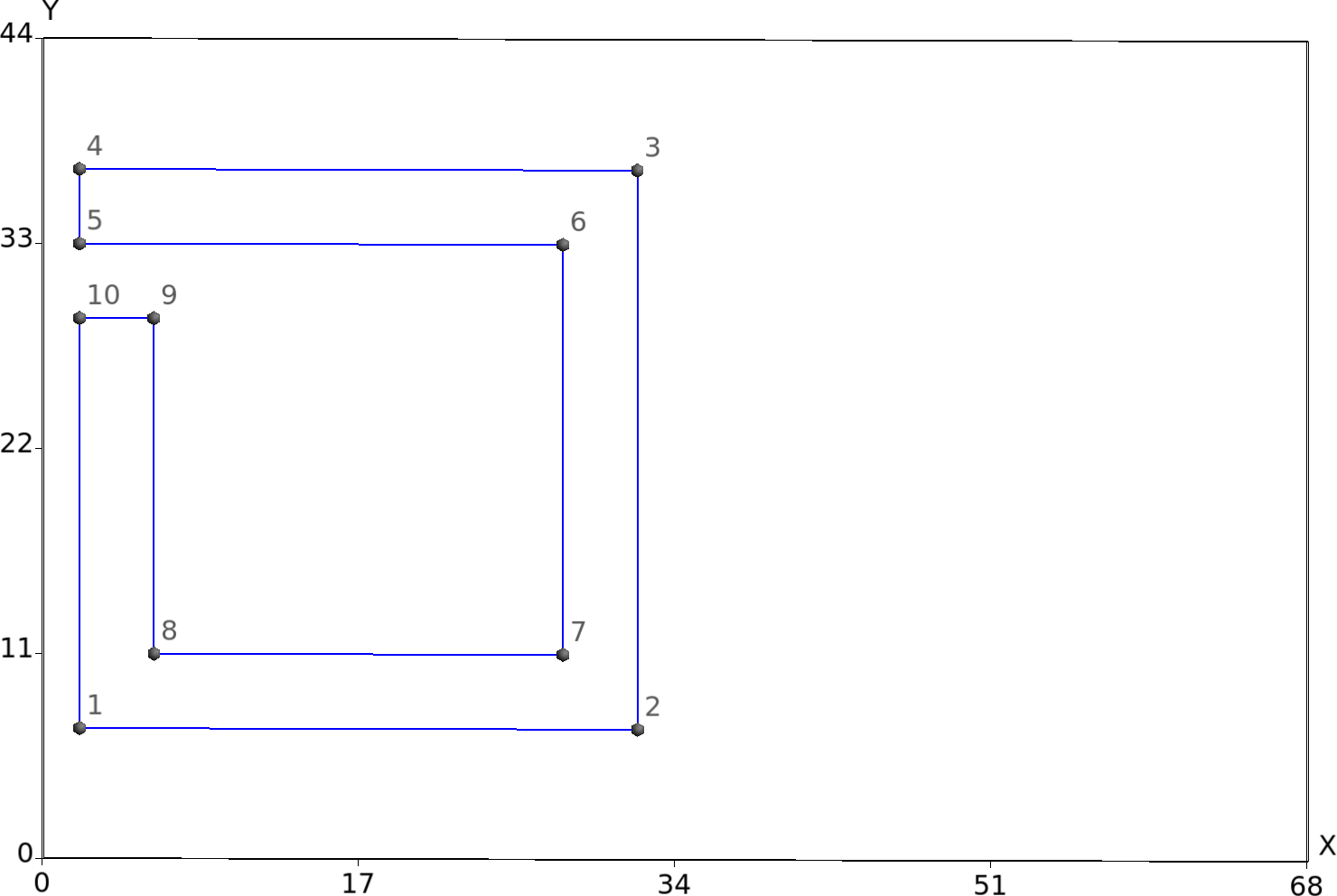SR.geo

DefineConstant [ T = 2 ];
DefineConstant [ W = 4 ];
DefineConstant [ Q = 14 ];
DefineConstant [ P = 22 ];
DefineConstant [ R = 24 ];
DefineConstant [ S = 22 ];
DefineConstant [ L = 1 ];

Point(1) = { S,   T,   0, L };
Point(2) = { S+R, T,   0, L };
Point(3) = { S+R, T+W, 0, L };
Point(4) = { S+W, T+W, 0, L };
Point(5) = { S+W, T+P-W, 0, L };
Point(6) = { S+R, T+P-W, 0, L };
Point(7) = { S+R, T+P+Q, 0, L };
Point(8) = { S,   T+P+Q, 0, L };
Point(9) = { S,   T+P+Q-W, 0, L };
Point(10) = { S+R-W,   T+P+Q-W, 0, L };
Point(11) = { S+R-W,   T+P, 0, L };
Point(12) = { S,   T+P, 0, L };

Line(1) = {1,2};
Line(2) = {2,3};
Line(3) = {3,4};
Line(4) = {4,5};
Line(5) = {5,6};
Line(6) = {6,7};
Line(7) = {7,8};
Line(8) = {8,9};
Line(9) = {9,10};
Line(10) = {10,11};
Line(11) = {11,12};
Line(12) = {12,1};

Line Loop(1) = {1,2,3,4,5,6,7,8,9,10,11,12};
Ruled Surface(1) = {1};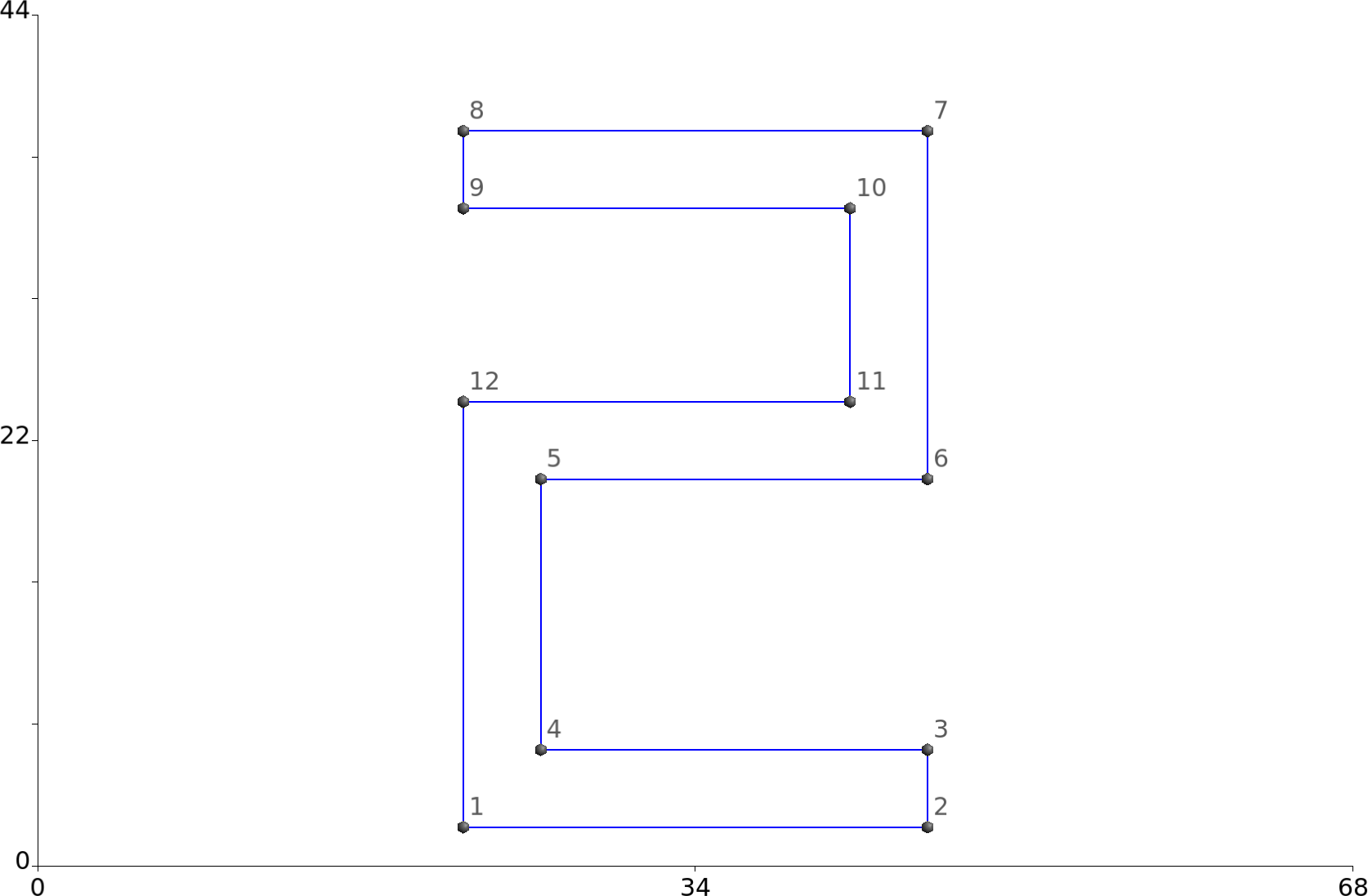### Create mesh files:

 % gmsh -2 ASRR.geo -o ASRR.msh
% gmsh -2 SR.geo -o SR.msh

ASRR.msh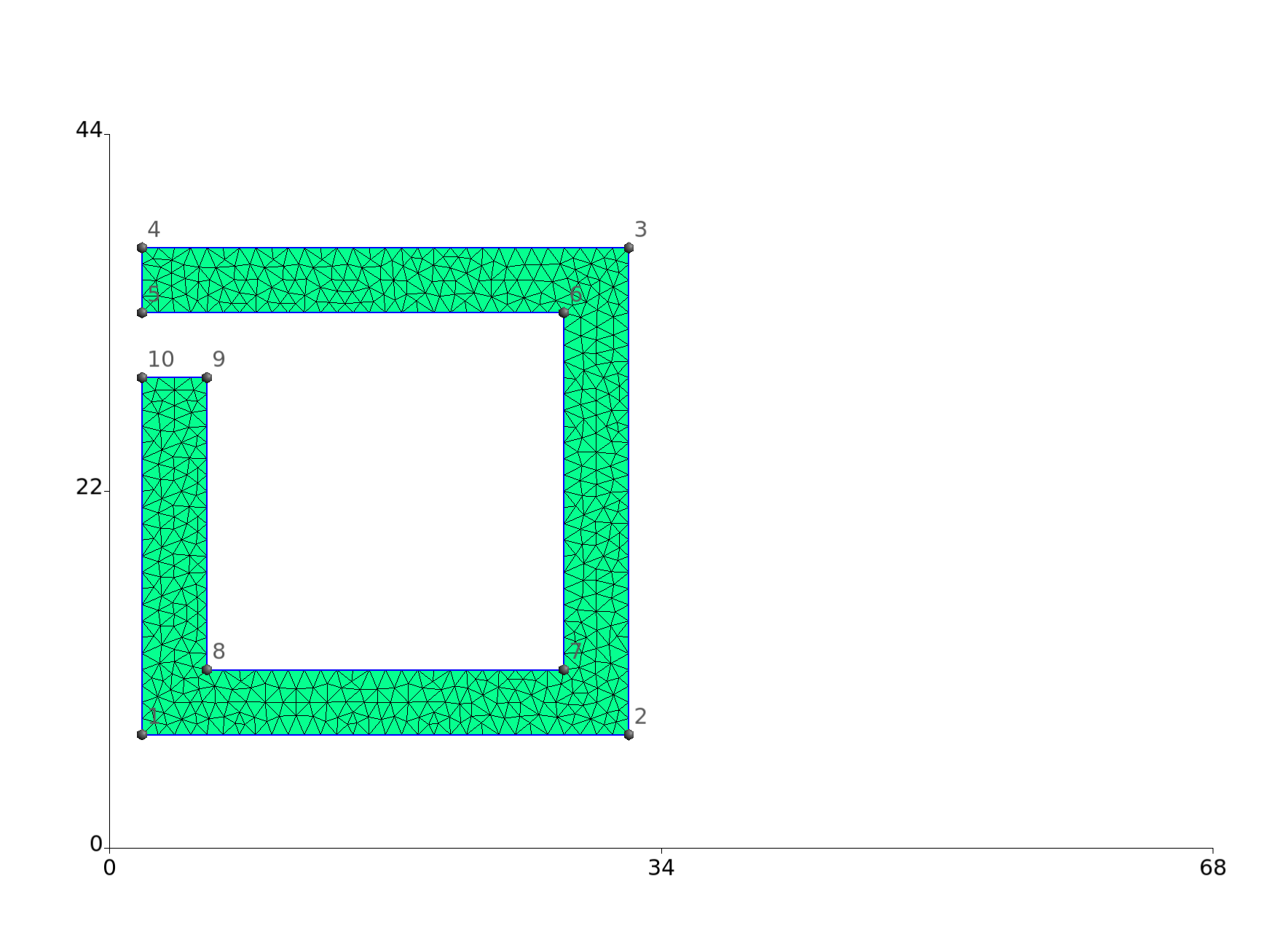SR.msh### SCUFF-EM geometry for periodic lattice of ASRRs / SRs

CMRFine.scuffgeo

LATTICE
VECTOR 68.0 0.0
VECTOR 0.0  44.0
ENDLATTICE

OBJECT LeftASRR
MESHFILE ASRR_1444.msh
DISPLACED 0 0 -25
ENDOBJECT

OBJECT RightASRR
MESHFILE ASRR_1444.msh
DISPLACED -17.0 -22.0 0.0
ROTATED 180 ABOUT 0.0 0.0 1.0
DISPLACED  17.0  22.0 0.0
DISPLACED 34.0 0.0 -25
ENDOBJECT

OBJECT SR
MESHFILE SR_1424.msh
DISPLACED 0.0 0.0 25
ENDOBJECT

### Use SCUFF-ANALYZE to obtain a visual image of the innermost several cells of the lattice

 % scuff-analyze --geometry CMRMedium.scuffgeo --WriteGMSHFiles --Neighbors 3
% Thank you for your support.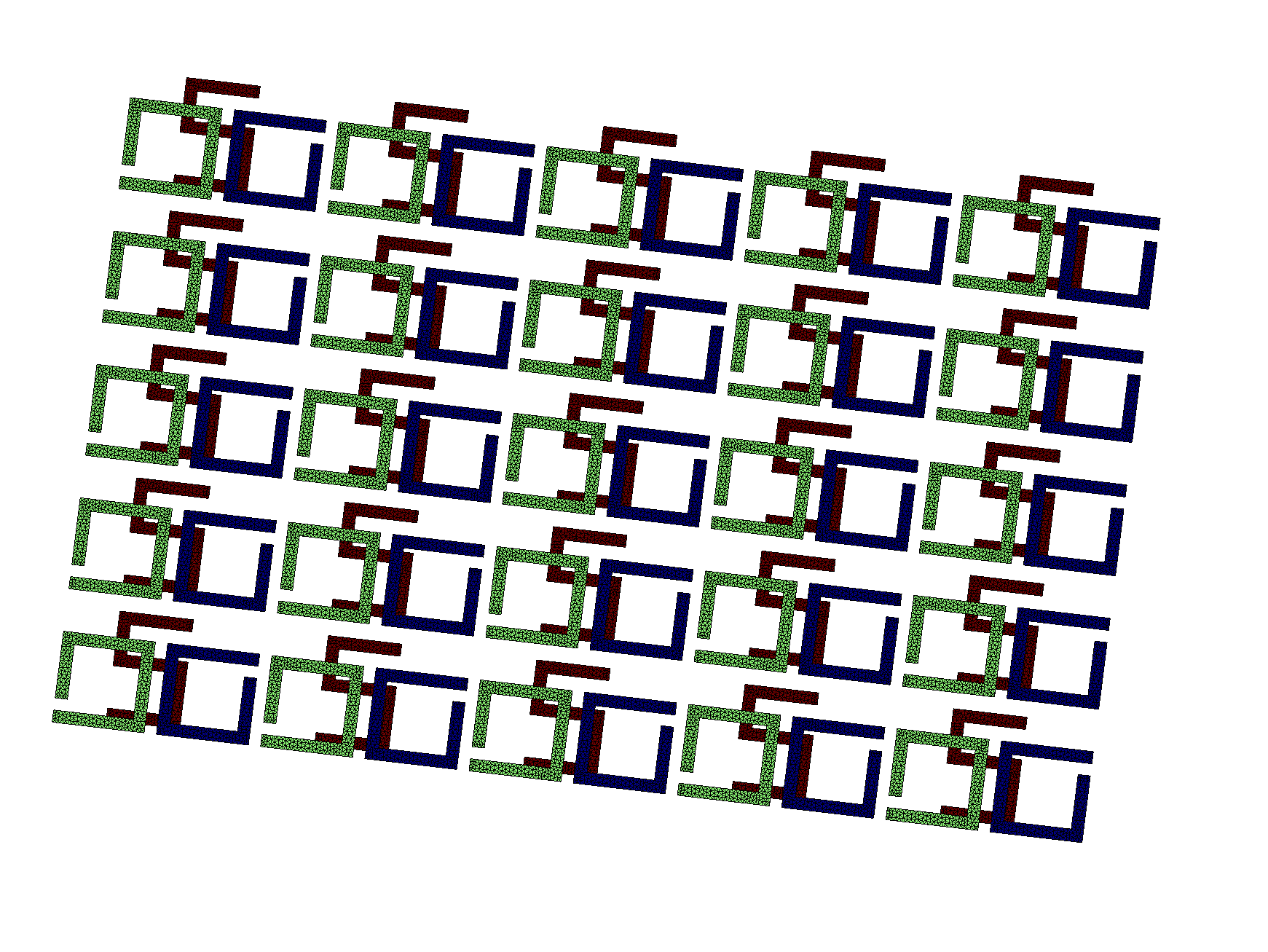### Use SCUFF-TRANSMISSION to compute transmission coefficients

 mylaptop% scuff-transmission --geometry CMRFine.scuffgeo --OmegaFile OmegaFile --ZAbove 25.0 --ZBelow -25.0

### Plot TE->TE and TE->TM transmission coefficients versus frequency in THz:

 gnuplot> THZ(w)=3e14*w/(2*pi*1e12)
gnuplot> plot 'CMRFine.transmission' u (THZ($1)):23 w lp pt 7 ps 1 t 'EX-EX', '' u (THZ($1)):25 w lp pt 6 ps 1.5 t 'EX-EY',